According to the kinetic theory of gases, at absolute temperature: [AIIM 1998; UPSEAT 2000]

(1) Water freezes                             (2) Liquid helium freezes

(3) Molecular motion stops               (3) Liquid hydrogen freezes

Concept Questions :-

Types of velocity
High Yielding Test Series + Question Bank - NEET 2020

Difficulty Level:

The figure shows two flasks connected to each other. The volume of the flask 1 is twice that of flask 2.  The system is filled with an ideal gas at temperature of 100 K and 200 K respectively.  If the mass of the gas in 1 be m then what is the mass of the gas in flask 2 [CBSE PMT 2000]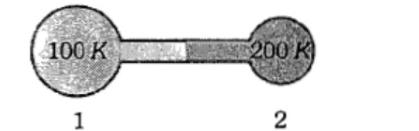(1) m              (2) m/2       (3) m/4        (4) m/8

Concept Questions :-

Ideal gas
High Yielding Test Series + Question Bank - NEET 2020

Difficulty Level:

PV versus T graph of equal masses of  is shown in the figure. Choose the correct alternative.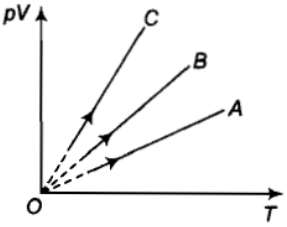1.  A corresponds to H2, B to He and C to O2

2.  A corresponds to He, B to H2 and C to O2

3.  A corresponds to He, B to O2 and C to H2

4.  A corresponds to O2, B to He  and C to H2

Concept Questions :-

Ideal gas
High Yielding Test Series + Question Bank - NEET 2020

Difficulty Level:

Which one of the following graphs represent the behaviour of an ideal gas at constant temperature?

(1)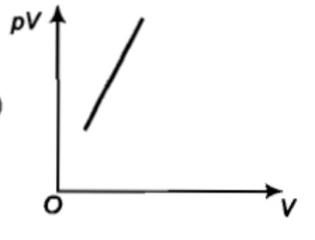(2)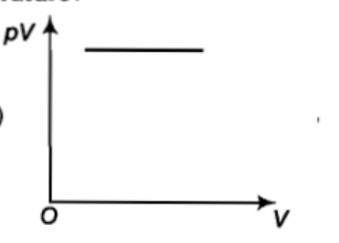(3)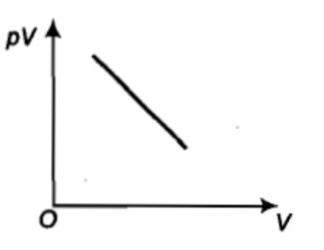(4)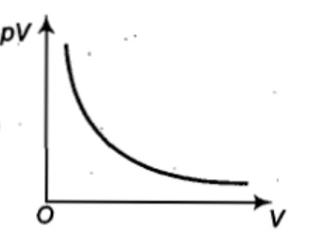Concept Questions :-

Ideal gas
High Yielding Test Series + Question Bank - NEET 2020

Difficulty Level:

The root-mean-square speed of hydrogen molecules at 300 K is 1930 m/s. Then the root mean square speed of oxygen molecules at 900 K will be: [MH CET 2002; Odisha JEE 2010]

(1) 1930$\sqrt{3}$ m/s                         (2) 836 m/s

(3) 643 m/s                                  (4) $\frac{1930}{\sqrt{3}}$ m/s

Concept Questions :-

Types of velocity
High Yielding Test Series + Question Bank - NEET 2020

Difficulty Level:

What is the mass of 2 liters of nitrogen at 22.4 atmospheric pressure and 273 K?

(1) 28 g               (2) 14$×22.4$ g

(3) 56 g               (4) None of these

Concept Questions :-

Ideal gas
High Yielding Test Series + Question Bank - NEET 2020

Difficulty Level:

Mean kinetic energy per degree of freedom of gas molecules is: [ MP PET 1995; RPET 1999, 2001, 03; Haryana CEET 2000; DCE 2009]

(1) $\frac{3}{2}kT$             (2) kT             (3) $\frac{1}{2}kT$             (4) $\frac{3}{2}RT$

Concept Questions :-

Law of equipartition of energy
High Yielding Test Series + Question Bank - NEET 2020

Difficulty Level:

An ideal gas is found to obey an additional law $V{P}^{2}$= constant. The gas is initially at temperature T and volume V. When it expands to a volume 2 V, the temperature becomes:

(1) T $\sqrt{2}$         (2) 2T         (3) 2T$\sqrt{2}$           (4) 4T

Concept Questions :-

Ideal gas
High Yielding Test Series + Question Bank - NEET 2020

Difficulty Level:

If the molecular weight of two gases are , then at a temperature the ratio of root mean square velocity  will be: [MP PMT 1996; AMU (Engg.) 2000; CPMT 2000; DPMT 2001]

(1) $\sqrt{\frac{{M}_{1}}{{M}_{2}}}$                          (2) $\sqrt{\frac{{M}_{2}}{{M}_{1}}}$

(3) $\sqrt{\frac{{M}_{1}+{M}_{2}}{{M}_{1}-{M}_{2}}}$                  (4) $\sqrt{\frac{{M}_{1}-{M}_{2}}{{M}_{1}+{M}_{2}}}$

Concept Questions :-

Types of velocity
High Yielding Test Series + Question Bank - NEET 2020

Difficulty Level:

An ideal gas is filled in a vessel, then

(1) If it is placed inside a moving train, its temperature increases

(2) Its centre of mass moves randomly

(3) Its temperature remains constant in a moving car

(4) None of these

Concept Questions :-

Kinetic energy of gas